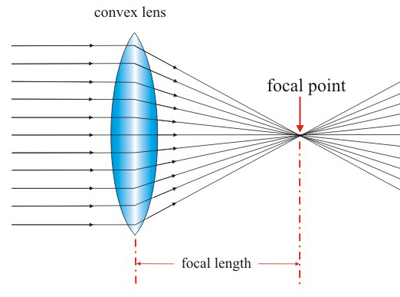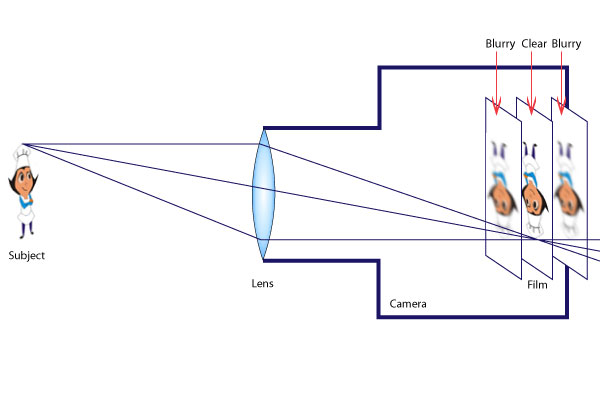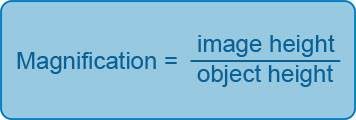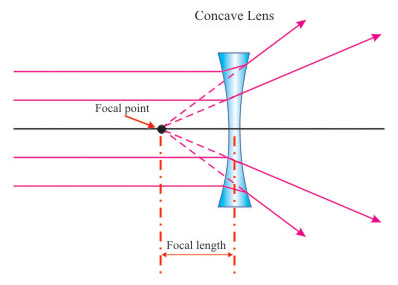Convex Lens

A convex lens is thicker at the centre than at the edges.Convex lenses are thicker at the middle. Rays of light that pass through the lens are brought closer together (they converge). A convex lens is a converging lens. When parallel rays of light pass through a convex lens the refracted rays converge at one point called the principal focus. The distance between the principal focus and the centre of the lens is called the focal length.

Use of Convex Lenses – The Camera

A camera consists of three main parts.

1. The body which is light tight and contains all the mechanical parts.
2. The lens which is a convex (converging) lens).
3. The film or a charged couple device in the case of a digital camera.The rays of light from the person are converged by the convex lens forming an image on the film or charged couple device in the case of a digital camera. The angle at which the light enters the lens depends on the distance of the object from the lens. If the object is close to the lens the light rays enter at a sharper angled. This results in the rays converging away from the lens. As the lens can only bend the light to a certain agree the image needs to be focussed in order to form on the film. This is achieved by moving the lens away from the film. Similarly, if the object is away from the lens the rays enter at a wider angle. This results in the rays being refracted at a sharper angle and the image forming closer to the lens. In this case the lens needs to be positioned closer to the film to get a focused image. Thus the real image of a closer object forms further away from the lens than the real image of a distant object and the action of focusing is the moving of the lens to get the real image to fall on the film. The image formed is said to be real because the rays of lighted from the object pass through the film and inverted (upside down).

The Magnifying Glass

A magnifying glass is a convex lens which produces a magnified (larger) image of an object.

A magnifying glass produces an upright, magnified virtual image. The virtual image produced is on the same side of the lens as the object. For a magnified image to be observed the distance between the object and the lens must be shorter than the focal length of the lens.A magnifying glass is a convex lens which produces a magnified image of an object.
 For a magnified image to be observed the distance between the object and the lens has to be shorter than the focal length of the lens. The image formed is upright, magnified and virtual.

Magnification

The magnification of a lens can be calculated using the following formula;As this is a ratio between heights it has no units. A magnification of 2 means the image is twice the size of the object and a magnification of 1 indicates an image size being the same as the object size.

Concave Lens

A concave lens is thinner at the centre than at the edges.Concave lenses are thinner at the middle. Rays of light that pass through the lens are spread out (they diverge). A concave lens is a diverging lens. When parallel rays of light pass through a concave lens the refracted rays diverge so that they appear to come from one point called the principal focus. The distance between the principal focus and the centre of the lens is called the focal length. The image formed is virtual and diminished (smaller)